April 30, 2019

Dimensionality Reduction with Principal Component Analysis

Principal component analysis (PCA) is an unsupervised machine learning algorithm used to reduce dimensions, in this post we will see how it works and how we can implement it using numpy.

Reduce dimensions can bring us some advantages like reduce the size needed to store the data, speed up training, improve accuracy and we can avoid problems like the Curse of dimensionality as well.

You need a lot of knowledge in linear algebra to understand this algorithm, however, I will try to keep it simple, we will see matrix multiplication, eigenvectors, eigenvalues, variance and covariance.

Firstly we need to remember how matrix multiplications work, we can see a matrix multiplication as a transformation where some matrix M is transforming a vector V in order to obtain a new transformed vector B.

When the matrix M transforms the vector V this matrix is transforming the space where the vector V lives as well, the eigenvectors are vectors which are not affected by this transformation, they only increase or decrease its size, in other words the eigenvectors change their length but not their direction. In the animation below we can see the transformation of the space and the blue arrows are the eigenvectors.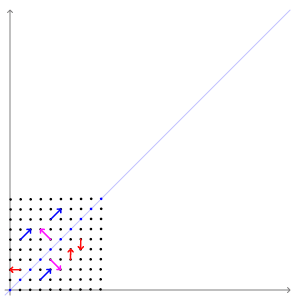We can notice that the eigenvectors tells us the direction where the space is transformed, in fact the eigenvectors of a matrix tell us the force that the matrix will apply to a vector.

Eigenvectors

We will see an example of eigenvectors in two different distributions: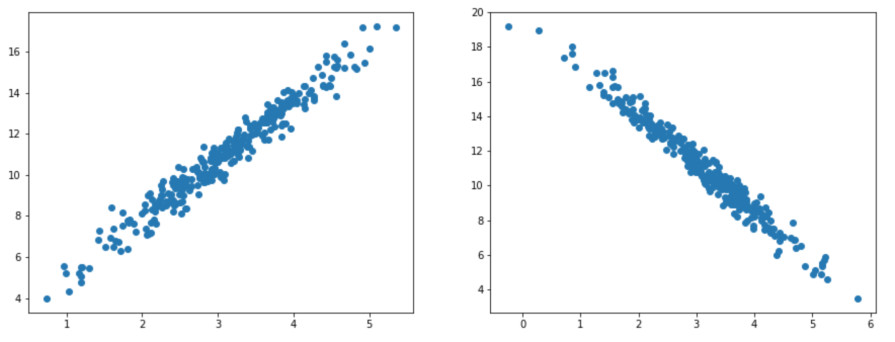The first distribution has a positive correlation and the second distribution has a negative correlation. If we compute the covariance matrices of these distributions we obtain the following matrices:

covariance_matrix_positive =
[[0.80333333, 2.26666667],
[2.26666667, 6.66666667]]

First distribution covariance matrix. The covariance is positive.

covariance_matrix_negative =
[[0.80333333, -2.26666667],
[-2.26666667, 6.66666667]]

Second distribution covariance matrix. The covariance is negative.

The numbers in the upper left and lower right are the variances of the X feature and the Y feature respectively, and the remaining identical numbers are the covariance between X and Y. Therefore, we can say that the variances are in the diagonal and the covariances in the off-diagonal. We could also say that the off-diagonal defines the orientation of the data, we can notice that when the covariance is positive the data goes right and up whereas when the covariance is negative the data goes right and down.

PCA draws straight lines like the linear regression model, we will have as many lines as many dimensions/features there are our data, the first line has the most variance in the data, and the posterior lines will take the remaining variance. We call these lines principal components.

Going back to our example, we can compute and plot the eigenvectors of our covariance matrices: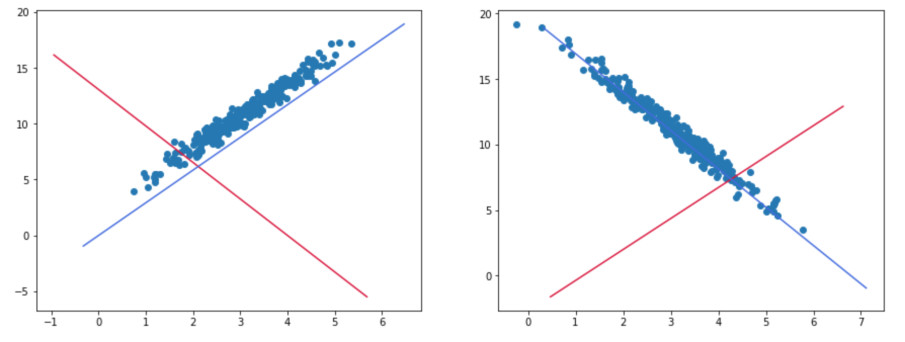We can notice that the blue eigenvector has the most variance in both distributions and the red eigenvector has the remaining variance, in fact PCA uses the eigenvectors of the covariance matrix to obtain the lines it needs.

As we previously saw the eigenvectors of a matrix tell us the force that the matrix will apply, if we use the covariance matrix with the variance and covariance of some dataset the eigenvectors of this matrix will tells us the force that was applied to this dataset, that's why we can use these eigenvectors to find out the maximum variance.

Eigenvalues

Each eigenvector has an eigenvalue, this eigenvalue tell us which eigenvector has the most variance in the dataset and the amount of variance as well.

If we want to obtain the percentage of variance of each principal component we divide each eigenvalue by the sum of all the eigenvalues, in the first example we obtained the following eigenvalues:

[7.44073168, 0.02926832]

If we sum both eigenvalues:

7.44073168 + 0.02926832 = 7.47

And we divide each eigenvalue:

7.44073168 / 7.47 = 0.9960818849
0.02926832 / 7.47 = 0.003918115127

We can see that almost all the variance is carried by the first principal component (the blue one).

Importance of Principal components

You may be wondering why we need these lines/vectors/principal components, in order to reduce dimensions PCA will project the data onto these lines, we will use a smaller dataset with the same variance and covariance that our positive distribution: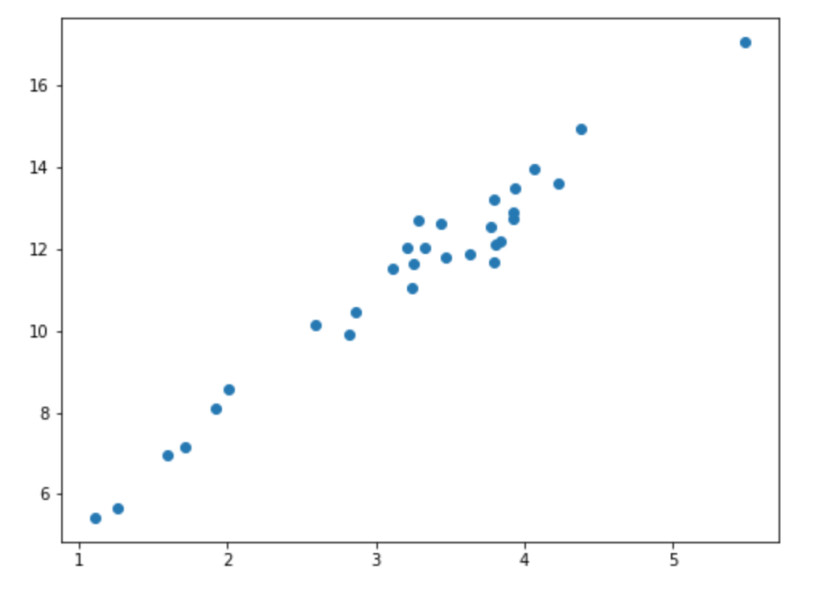If we compute the eigenvectors and use the one with the greatest eigenvalue: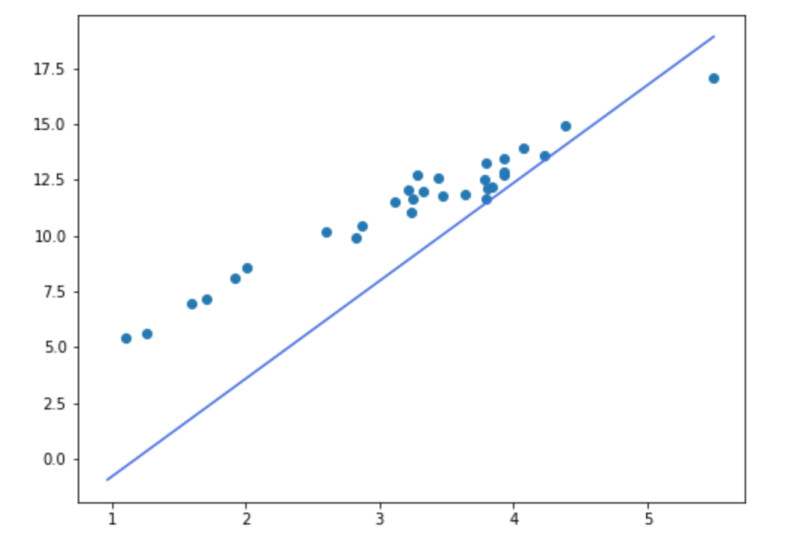PCA will project the data onto this principal component to remove one dimension: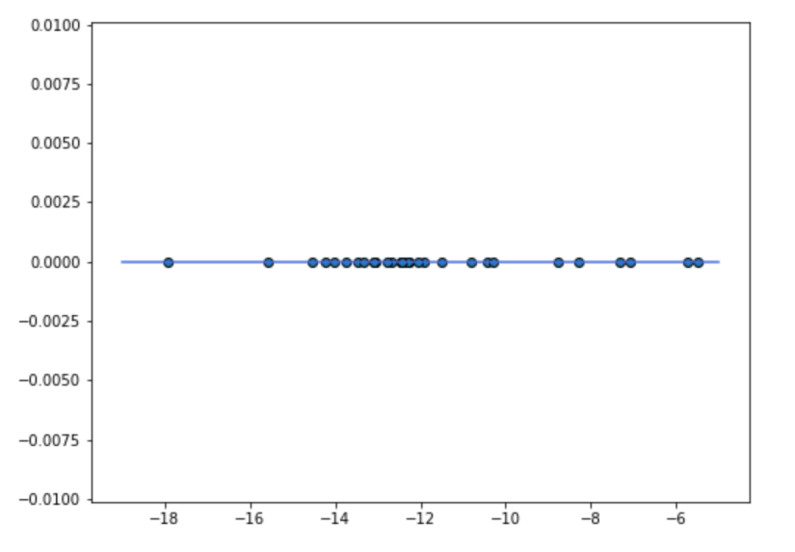We can see that the range of this dataset goes from -19 to -7 and the variance is 7.7.

If we use a random vector like the one below: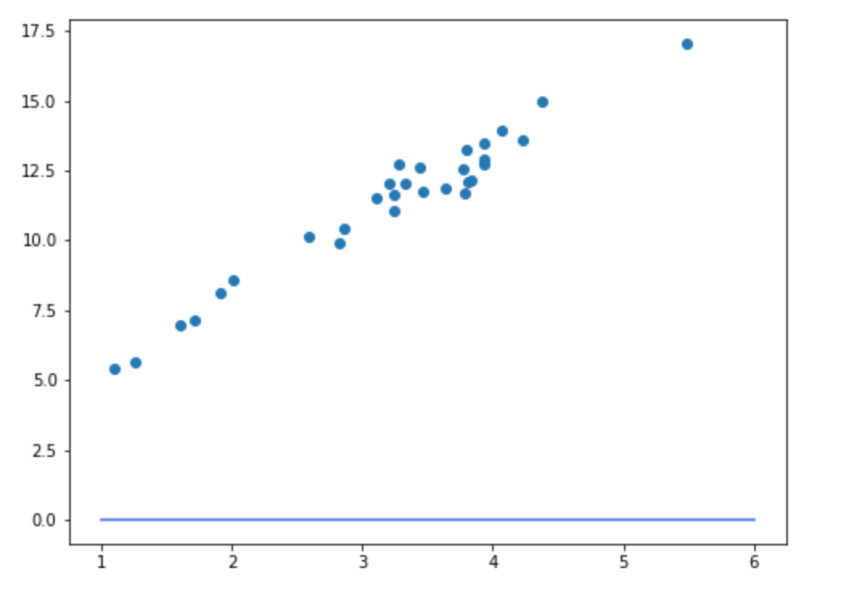When PCA projects the data onto this principal component we will obtain the following result: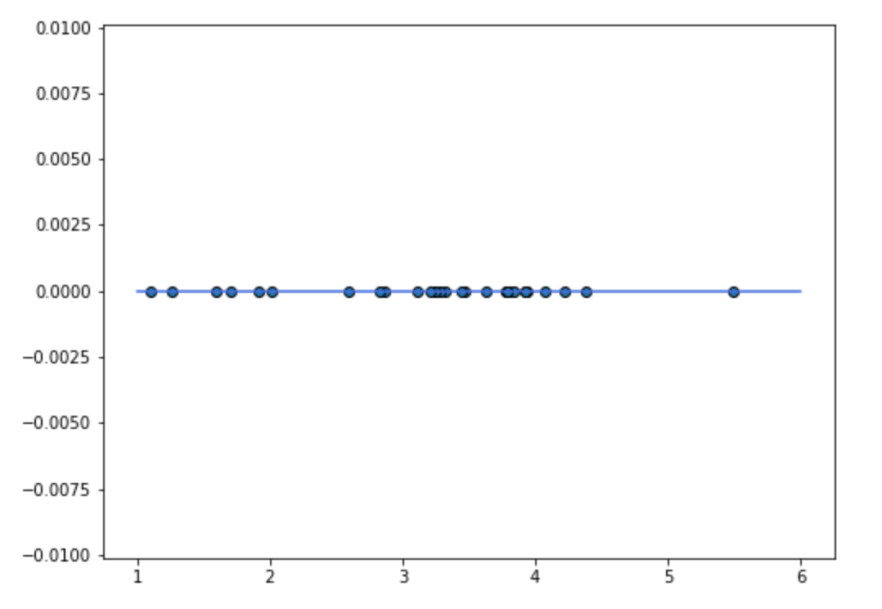Perhaps is hard to see a difference, however, this time the range goes from 1 to 6 and if we compute the variance we obtain a value of 0.95. Therefore, the only way to obtain the greatest variance is using the eigenvectors.

Iris Dataset

We will use numpy to implement PCA and reduce one dimension of the iris dataset, this dataset has 4 dimensions but we will use only 2, we can plot these two dimension as follows: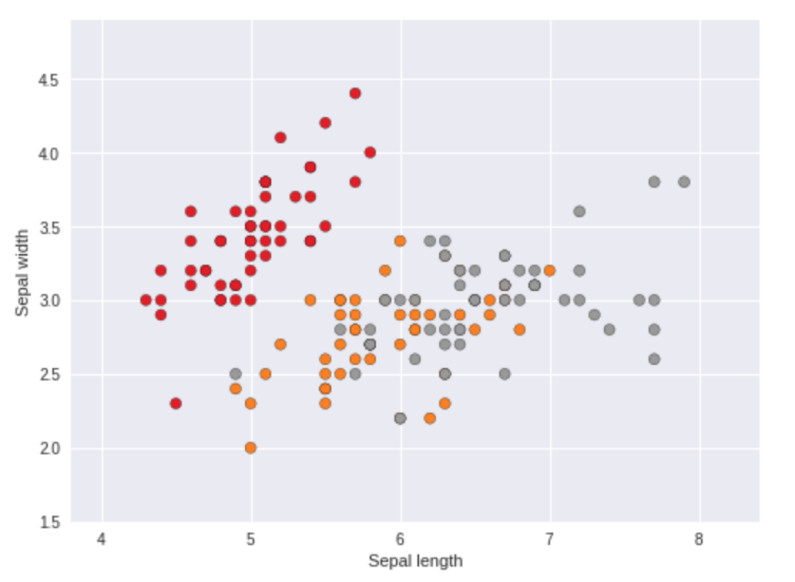The first thing we need to do is load and normalize the dataset

X = iris.data[:, :2]
y = iris.target

normalized_x = StandardScaler().fit_transform(X)

As we have seen in previously posts there are features that can have values between 0-100 and others that can have values between 0-1, the variance of the features with larger ranges is greater than the variance of features with smaller ranges, this can affect our PCA model and we could end up with biased results, in order to avoid this we normalize all the features to have the same range between 0-1.

To obtain the eigenvectors we need to compute the covariance matrix:

covariance_matrix = np.cov(normalized_x.T)

Now we can obtain the eigenvectors of the covariance matrix:

eigen_values, eigen_vectors = np.linalg.eig(covariance_matrix)

We can plot the eigenvectors: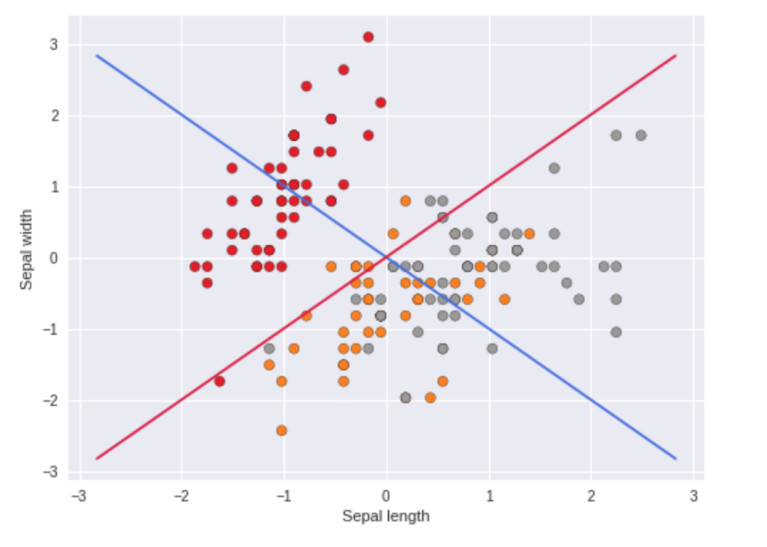The blue principal component has the most variance in the data and the red principal component has the remaining variance.

Once we have the principal components of the covariance matrix PCA will use them as the new axes of the dataset:

transformed_data = np.dot(eigen_vectors.T, normalized_x.T)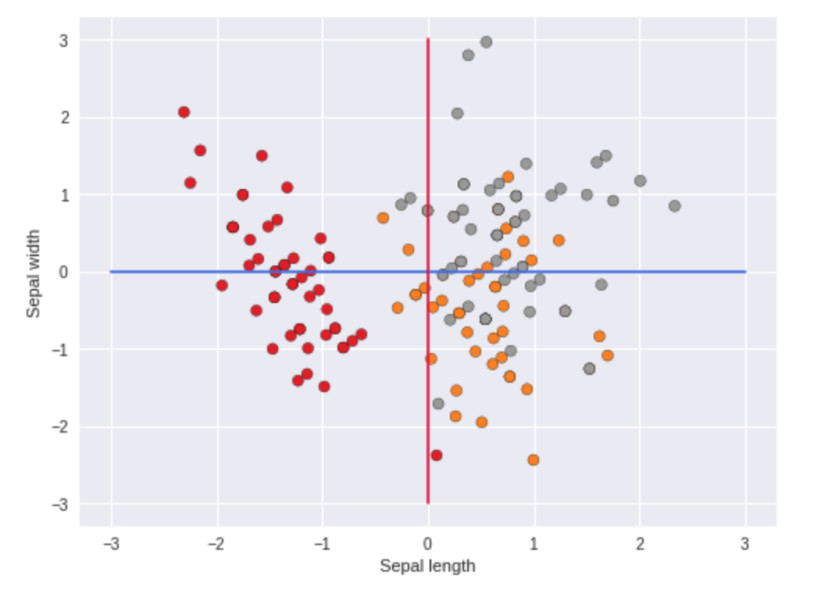We can notice that the matrix multiplication rotated the data but we kept both dimensions, if we want to reduce one dimension we have to select the principal component with the greatest eigenvalue and project the data onto that component.

principal_component = eigen_vectors.reshape(1, 2)
one_dimension_data = np.dot(principal_component, x_std.T)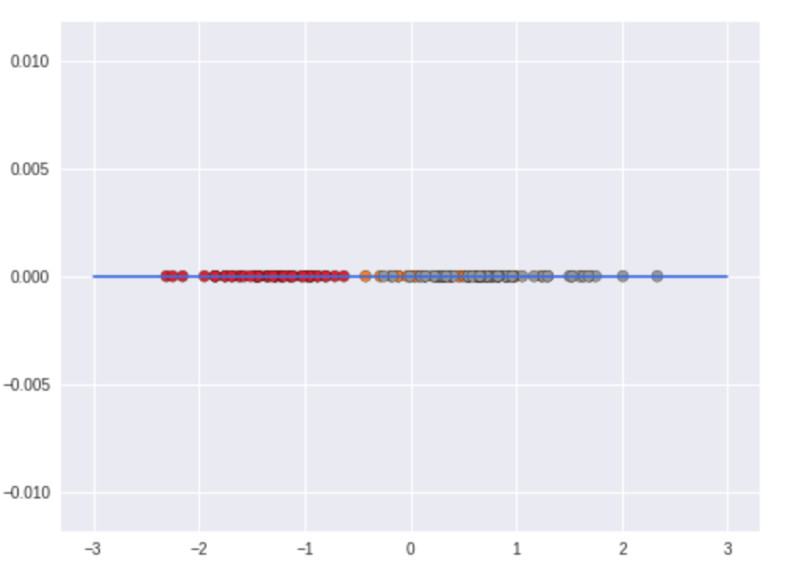This time we only have one dimension since we projected all the data onto the principal component.

PCA is very usefully when we have a dataset with a big amount of dimensions and we want to reduce those dimension to plot the data or to reduce the training time and increase the accuracy of some model.

One of the problems of this model is the fact that we loss the interpretability of the dataset, if we have two features sepal width and sepal length once we apply the PCA algorithm we will loss these features and obtain one or two new features, these new features have different interpretations.